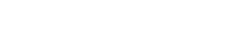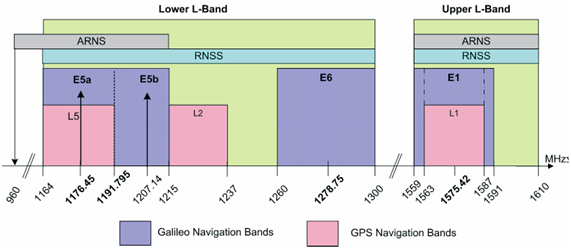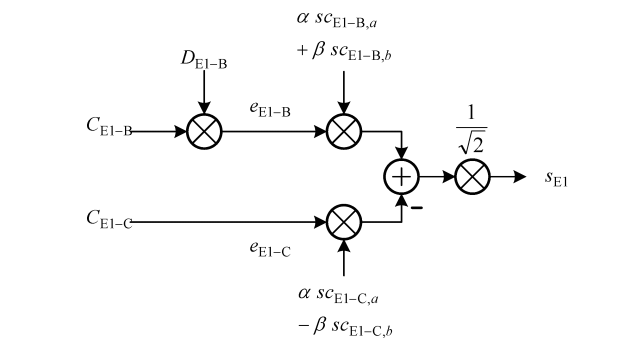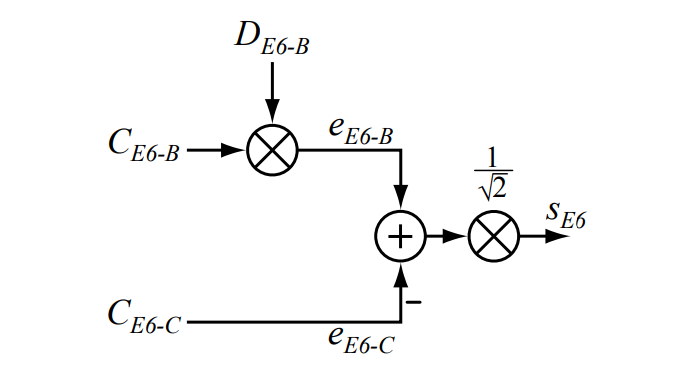If you wish to contribute or participate in the discussions about articles you are invited to contact the Editor

# Galileo Signal PlanGALILEO
Title Galileo Signal Plan
Author(s) J.A Ávila Rodríguez, University FAF Munich, Germany.
Year of Publication 2011

Galileo satellites transmit permanently three independent CDMA and Right-Hand Circularly Polarised (RHCP) signals, named E1, E5 and E6. The E5 signal is further sub-divided into signals denoted E5a and E5b. These signals are transmitted in four frequency bands which provide a wide bandwidth for the transmission of the Galileo signals, as seen in figure 1: Galileo Frequency plan. Table 1 specifies the corresponding carrier frequencies and receiver reference bandwidths for the Galileo signals.Figure 1: Galileo Frequency Plan, Credits: Galileo OS SIS ICD
Table 1: Corresponding carrier frequencies and receiver reference bandwidths for the Galileo signals.
Signal Carrier Frequency (MHz) Receiver Reference Bandwidth (MHz)
E1 1575.420 24.552
E6 1278.750 40.920
E5 1191.795 51.150
E5a 1176.450 20.460
E5b 1207.140 20.460

The Galileo frequency bands have been selected in the allocated spectrum for Radio Navigation Satellite Services (RNSS) and in addition to that, E5a, E5b and E1 bands are included in the allocated spectrum for Aeronautical Radio Navigation Services (ARNS), employed by civil aviation users and allowing dedicated safety-critical applications.

## Galileo E1 Band

The E1 Open Service (OS) modulation receives the name of CBOC (Composite Binary Offset Carrier) and is a particular implementation of MBOC (Multiplexed BOC) [J.-A. Avila-Rodriguez et al., 2007]. MBOC(6,1,1/11) is the result of multiplexing a wideband signal – BOC(6,1) – with a narrowband signal – BOC(1,1) – in such a way that 1/11 of the power is allocated, in average, to the high frequency component. This signal was the last one to be defined.

The normalized (unit power) power spectral density, specified without the effect of band-limiting filters and payload imperfections, is given by

The generic view of the E1 Open Service signal generation can be depicted as follows:Figure 1: Modulation Scheme for the Galileo E1 CBOC Signal, Credits: Galileo OS SIS ICD.

The whole transmitted Galileo E1 signal consists of the multiplexing of the following components:

• The E1 Open Service Data channel [math]e_{E1-B}(t)[/math] is generated from the I/NAV navigation data stream [math]D_{E1-B}(t)[/math] and the ranging code [math]C_{E1-B}(t)[/math], which are then modulated with the sub-carriers [math]SC_{E1-B,a}(t)[/math] and [math]SC_{E1-B,b}(t)[/math] respectively.
• The E1 Open Service Pilot channel [math]e_{E1-C}(t)[/math] is generated from the ranging code [math]C_{E1-C}(t)[/math], including its secondary code, which is then modulated with the sub-carriers [math]SC_{E1-C,a}(t)[/math] and [math]SC_{E1-C,b}(t)[/math] in anti-phase.

For more details on the mathematical definition of the signal please refer to Coherent Adaptive Sub-Carrier Modulation (CASM) and Interplex where it is described more in detail.

It is interesting to see how the spectra of the two systems described so far in E1/L1 overlap:

It is important to recall that for a long time the actual E1 band received the name of L1 band in analogy with GPS and it was not until the publication of the version of Galileo OS SIS ICD published in 2008 [Galileo SIS ICD, 2008]  that L1 changed to the current E1.

The E1 Open Service (OS) codes are, as well as the E6 CS codes that we will see later, also random memory codes. The plain number of choices to set the 0’s and 1’s for the whole code family is enormous and thus special algorithms have to be applied to generate random codes efficiently [J.-A. Avila-Rodriguez et al., 2007] .

Finally, the technical characteristics of all the Galileo signals in E1 can be summarized in the following table:

## Galileo E6 Band

Two dedicated signals are transmitted on the E6 band (1260-1300 MHz): a data component (E6-B) component allowing the transmission of 448 bits per second and a pilot (E6-C) component. Both channels allow to encrypt the information at signal level. E6 signals are modulated with a binary phase shift keying BPSK(5) at a carrier frequency of 1278.75 MHz, which is used by all satellites and shared though a CDMA RF channel access mode.

Therefore, as shown in Galileo OS SIS ICD, the transmitted Galileo E6 signal consists of the following components, both (pilot and data components) are combined on the same carrier component, with a power sharing of 50 percent:

• [math]e_{E6-B}[/math] from the C/NAV navigation data stream [math]D_{E6-B}[/math] modulated with the encrypted ranging code [math]C_{E6-B}[/math]
• [math]e_{E6-C}[/math] (pilot component) from the ranging code [math]C_{E6-C}[/math]

This is graphically shown as follows:Figure 4: Modulation Scheme for the Galileo E6 Signals, Credits: Galileo OS SIS ICD

Moreover, the spectrum of the different E6 signals is shown to be as follows:

The E6 Commercial Service (CS), now High Accuracy Service (HAS), codes are random codes [J. Winkel, 2006] . The main idea behind is to generate a family of codes that fulfils the properties of randomness as well as possible [J.-A. Avila-Rodriguez et al., 2007] . The codes can be driven to fulfil special properties such as balance and weakened balance, where the probability of 0’s and 1’s must not be identical but within a well-defined range, or to realize the autocorrelation side-lobe zero (ASZ) property. This latter property guarantees that the autocorrelation values of every code correlate to zero with a delayed version of itself, shifted by one chip. The main specifications of the Galileo E6-b and E6-C codes to facilitate the development of Galileo E6-B/C enabled receivers have already been published.

## Galileo E5 Band

The different Galileo E5 signal components are generated according to the Galileo OS SIS ICD:

• [math]e_{E5a-I}[/math] from the F/NAV navigation data stream [math]D_{E5a-I}[/math] modulated with the unencrypted ranging code [math]C_{E5a-I}[/math]
• [math]e_{E5a-Q}[/math] (pilot component) from the unencrypted ranging code [math]C_{E5a-Q}[/math]
• [math]e_{E5b-I}[/math] from the I/NAV navigation data stream [math]D_{E5b-I}[/math] modulated with the unencrypted ranging code [math]C_{E5b-I}[/math]
• [math]e_{E5b-Q}[/math] (pilot component) from the unencrypted ranging code [math]C_{E5b-Q}[/math]

The E5 modulation receives the name of AltBOC and is a modified version of a Binary Offset Carrier (BOC) with code rate of 10.23 MHz and a sub-carrier frequency of 15.345 MHz. AltBOC(15,10) is a wideband signal that is transmitted at 1191.795 MHz. Next figure shows the Galileo E5 signal modulation diagram:

The E5 signal modulation spectrum adopts the following form:

As we can recognize from the figure above, the AltBOC(15,10) modulation is very similar to two BPSK(10) signals shifted by 15 MHz to the left and right of the carrier frequency. Indeed, since to acquire all the main lobes of the modulation a very wide bandwidth is necessary, many receivers will operate correlating the AltBOC signal with a BPSK(10) replica.

To have a better feeling about the overlapping between GPS and Galileo in E5, the next figure shows all the signals described so far for this band.

The E5 primary codes can be generated with shift registers. Indeed, the outputs of two parallel registers are modulo-two added to generate the primary codes. For more details on the start values of the primary codes and the corresponding secondary codes of each satellite, refer to Galileo OS SIS ICD. Finally, some details on the technical characteristics of the E5 signal are presented.

## Galileo C-band

The Radio-Navigation Satellite Service (RNSS) portion of the RF spectrum is overcrowded. This is especially true on the E1/L1 band. Nevertheless even those bands that have not been used yet will certainly be shared by many systems in the near future. Thus, the search of other free frequency resources is something that will occur with a high probability in the next years.

During the World Radio Conference 2000 (WRC-2000), the Galileo program obtained authorization to use C-band frequencies between 5010 and 5030 MHz. At the time, a dedicated portion of the C-band was assigned for Radio-Navigation, but technical complexities made it impossible for the first generation of Galileo.

Indeed, phase noise problems, the higher free space attenuation (related to the use of omni directional antennae) and the strong signal attenuation due to rain made all the proposed solutions not adequate for the first Galileo constellation. However, in some decades things could have changed and C-band could be a real alternative. As suggested in [G.W. Hein et al., 2007b]  and [G.W. Hein et al., 2007c], the C-band could be reserved for military/governmental applications leaving the L-band alone for civil users. This would have interesting benefits to both types of user and consequences as depicted in that article.